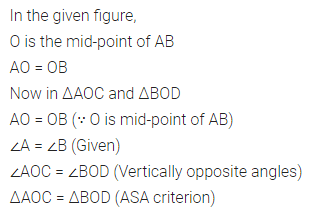# ML Aggarwal Class 7 Solutions for ICSE Maths Chapter 12 Congruence of Triangles Ex 12.2

## ML Aggarwal Class 7 Solutions for ICSE Maths Chapter 12 Congruence of Triangles Ex 12.2

Question 1.
You want to establish ΔDEF = ΔMNP, using ASA rule of congruence. You are given that ∠D = ∠M and ∠F = ∠P. What additional information is needed to establish the congruence?
Solution: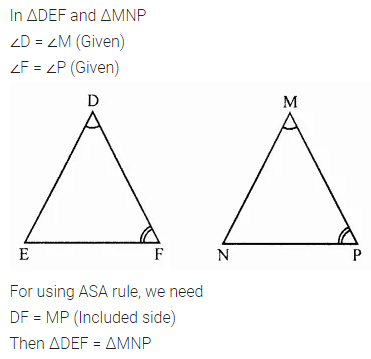Question 2.
In the given figure, two triangles are congruent. The corresponding parts are marked. We can write ΔRAT =?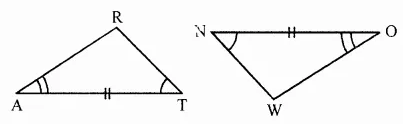Solution:Question 3.
If ΔABC and ΔPQR are to be congruent, name one additional pair of corresponding parts. What criterion did you use?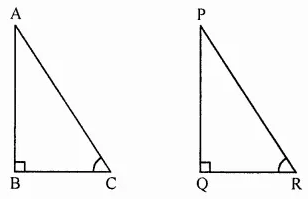Solution:Question 4.
Given below are measurements of some parts of two triangles. Examine whether the two triangles are congruent or not, by ASA congruence rule. In the case of congruence, write its in symbolic form.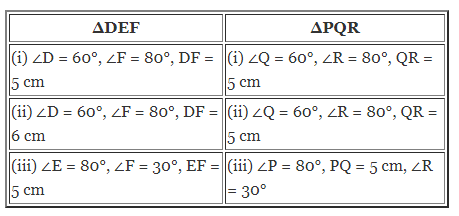Solution: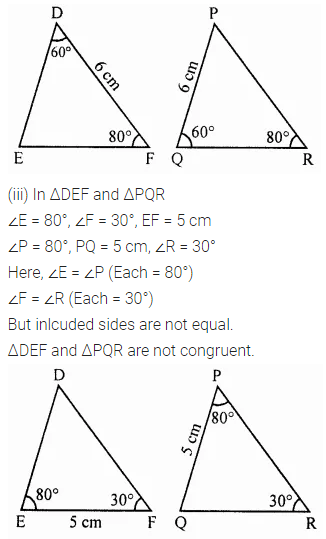Question 5.
In the adjoining figure, measures of some parts are indicated.
(i) State three pairs of equal parts in triangles ABC and ABD.
(ii) Is ΔABC = ΔBAD? Give reasons.
(iii) Is BC = AD? Why?Solution:Question 6.
In the adjoining figure, ray AZ bisects ∠DAB as well as ∠DCB.
(i) State the three pairs of equal parts in triangles BAC and DAC.
(ii) Is ΔBAC = ΔDAC? Give reasons.
(iii) Is CD = CB? Give reasons.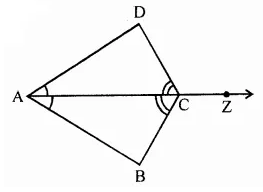Solution:Question 7.
Explain why ΔABC = ΔFED?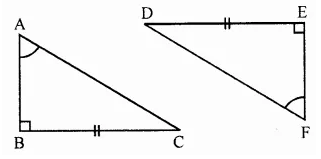Solution: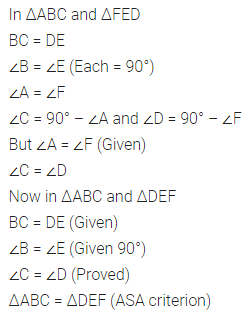Question 8.
Given below are the measurements of some parts of triangles. Examine whether the two triangles are congruent or not, using RHS congruence rule. In the case of congruent triangles, write the result in symbolic form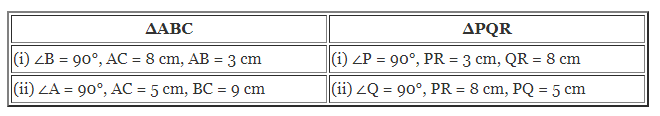Solution: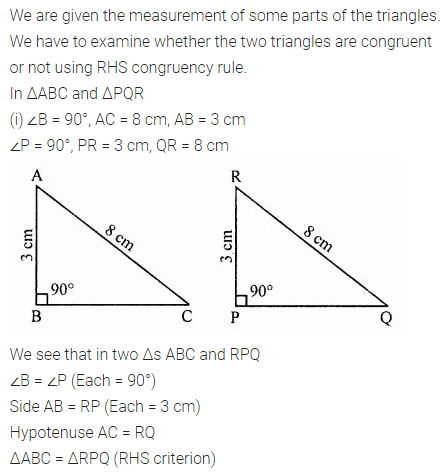Question 9.
In the given figure, measurements of some parts are given.
(i) State the three pairs of equal parts in ΔPQS and ΔPRS.
(ii) Is ΔPQS = ΔPRS? Give reasons.
(iii) Is S mid-point of $$\bar { QR }$$ ? Why?Solution: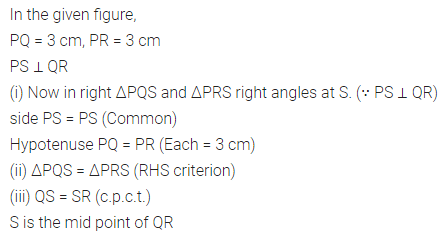Question 10.
In the given figure, O is mid-point of $$\bar { AB }$$ and ∠A = ∠B. Show that ΔAOC = ΔBOD.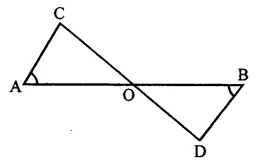Solution: